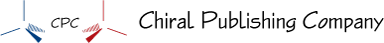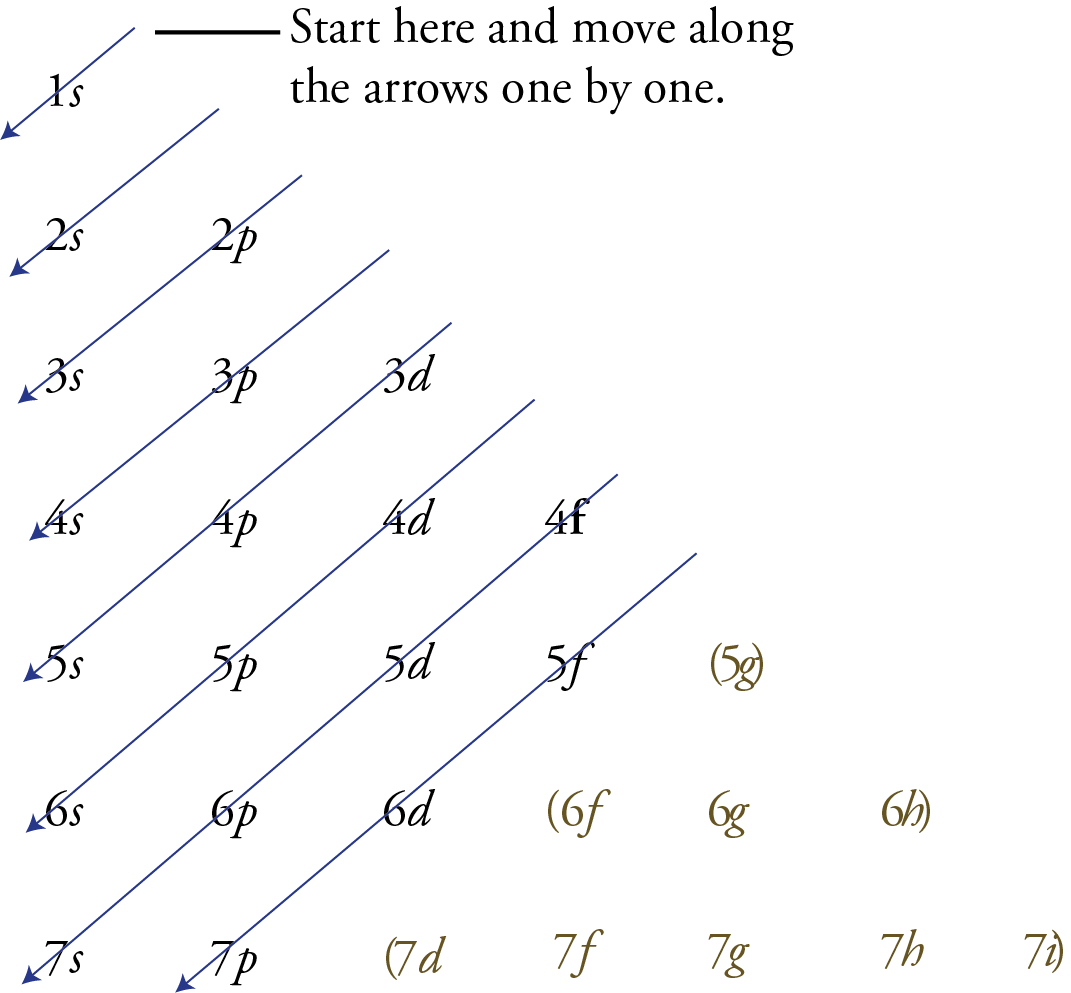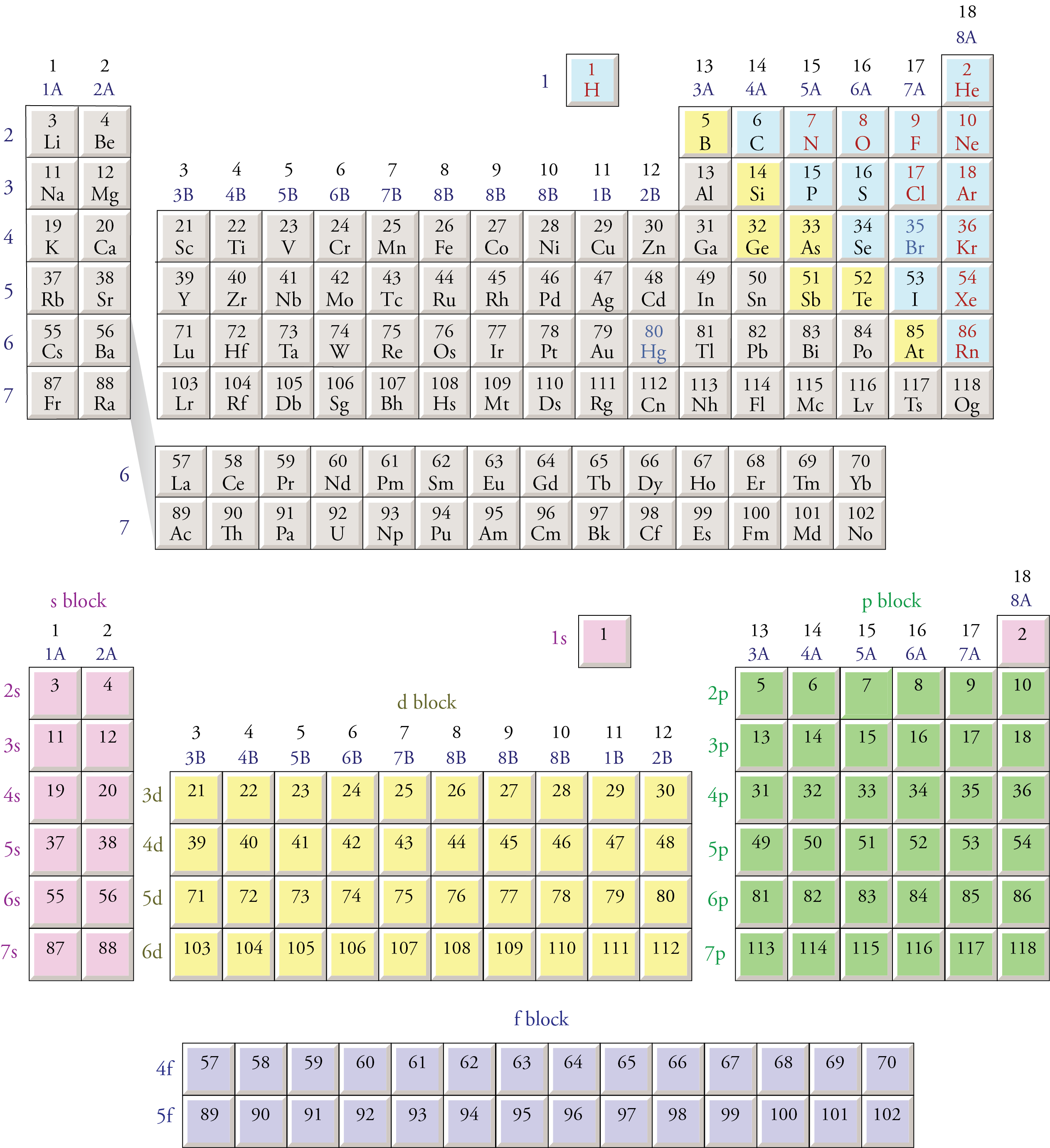﻿ Electron configurations

#Complete Electron Configurations

• To write a complete electron configuration for an uncharged atom,

Determine the number of electrons in the atom from its atomic number. (See Below.)

Add electrons to the sublevels in the correct order of filling.

Add two electrons to each s sublevel, 6 to each p sublevel, 10 to each d sublevel, and 14 to each f sublevel.

To check your complete electron configuration, look to see whether the location of the last electron added corresponds to the element’s position on the periodic table.

Predicting the Order of Filling of the Orbitals

There are three ways to predict the order of filling of the orbitals. Probably the least reliable method is to memorize the following list (even though it shows the order of filling of all the orbitals necessary for describing the ground state electron configurations of all of the known elements).

1s 2s 2p 3s 3p 4s 3d 4p 5s 4d 5p 6s 4f 5d 6p 7s 5f 6d 7p

Instead of relying on memorization, you can use the memory aid shown below to remind you of the correct order of filling of the sublevels. The following steps explain how to write it and use it yourself.

• Write the possible sublevels for each energy level in organized rows and columns like the image below. To do this, you need to remember that there is one sublevel on the first principal energy level, two on the second, three on the third, etc. Every principal energy level has an s orbital. The second principal energy and all higher energy levels have a p sublevel. The d sublevels start on the third principal energy level, the f sublevels start on the fourth principal energy level, etc.

• Draw arrows like those you see below .

• Starting with the top arrow, follow the arrows one by one in the direction they point, listing the sublevels as you pass through them.

• The sublevels that are not needed for describing the known elements are enclosed in parentheses below .We can also use the block organization of the periodic table, as shown below, to remind us of the order in which sublevels are filled. To do this, we move through the elements in the order of increasing atomic number, listing new sublevels as we come to them. The type of sublevel (s, p, d, or f ) is determined from the block in which the atomic number is found. The number for the principal energy level (for example, the 3 in 3p) is determined from the row in which the element is found and the knowledge that the s sublevels start on the first principal energy level, the p sublevels start on the second principal energy level, the d sublevels start on the third principal energy level, and the f sublevels start on the fourth principal energy level.

We know that the first two electrons added to an atom go to the 1s sublevel.

Atomic numbers 3 and 4 are in the second row of the s block (look for them in the bottom half of in image below), signifying that the 3rd and 4th electrons are in the 2s sublevel.

Atomic numbers 5 through 10 are in the first row of the p block, and the p sublevels start on the second energy level. Therefore, the 5th through 10th electrons go into the 2p sublevel.

Atomic numbers 11 and 12 are in the third row of the s block, so the 11th and 12th electrons go into the 3s sublevel.

Because atomic numbers 13 through 18 are in the p block, we know they go into a p sublevel. Because the p sublevels begin on the second principal energy level and atomic numbers 13 through l8 are in the second row of the p block, the 13th through 18th electrons must go into the 3p sublevel.

The position of atomic numbers 19 and 20 in the fourth row of the s block and the position of atomic numbers 21 through 30 in the first row of the d block show that the 4s sublevel fills before the 3d sublevel.

Moving through the periodic table in this fashion produces the following order of sublevels up through 6s:

1s 2s 2p 3s 3p 4s 3d 4p 5s 4d 5p 6s

Notice that atomic numbers 57 through 70 on the periodic table below are in the 4f portion of the table. It is a common mistake to forget that the 4f sublevel is filled after the 6s sublevel and before the 5d sublevel. In order to make the overall shape of the table more compact and convenient to display, scientists have adopted the convention of removing the elements with atomic number 57 through 70 and 89 through 102 (the latter being the 5f portion of the table) from their natural position between the s and d blocks and placing them at the bottom of the table. Electrons go into the 5f sublevel after the 7s sublevel and before the 6d sublevel. The second periodic table below shows how the blocks on the periodic table would fit together if the inner transition metals­—the f block—were left in their natural position.Chiral Publishing Company